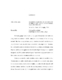## HIERARCHICAL BAYES ESTIMATION AND EMPIRICAL BEST PREDICTION OF SMALL-AREA PROPORTIONS

 dc.contributor.advisor Lahiri, Partha en_US dc.contributor.author Liu, Benmei en_US dc.contributor.department Survey Methodology en_US dc.contributor.publisher Digital Repository at the University of Maryland en_US dc.contributor.publisher University of Maryland (College Park, Md.) en_US dc.date.accessioned 2009-07-02T05:41:26Z dc.date.available 2009-07-02T05:41:26Z dc.date.issued 2009 en_US dc.description.abstract Estimating proportions of units with a given characteristic for small areas using small area estimation (SAE) techniques is a common problem in survey research. The direct survey estimates, usually based on area-specific sample data, are very imprecise or even unavailable due to the small or zero sample sizes in the areas. In order to provide precise estimates, a variety of model-dependent techniques, using Bayesian and frequentist approaches, have been developed. Among those, empirical best prediction (EBP) and hierarchical Bayes (HB) methods relying on mixed models have been considered for estimating small area proportions. Mixed models can be broadly classified as area or unit level models in SAE. When an area level model is used to produce estimates of proportions for small areas, it is commonly assumed that the survey weighted proportion for each sampled small area has a normal distribution and that the sampling variance of this proportion is known. However, these assumptions are problematic when the small area sample size is small or when the true proportion is near 0 or 1. In addition, normality is commonly assumed for the random effects in area level and unit level mixed models. However, this assumption maybe violated for some cases. To address those issues, in this dissertation, we first explore some alternatives to the well-known Fay-Herriot area level model. The aim is to consider models that are appropriate for survey-weighted proportions and can capture different sources of uncertainty, including the uncertainty that arises from the estimation of the sampling variances of the design-based estimators. Then we develop an adaptive HB method for SAE using data from a simple stratified design. The main goal is to relax the usual normality assumption for the random effects and instead determine the distribution of the random effects adaptively from the survey data. The Jiang-Lahiri type frequentist's alternative to the hierarchical Bayesian methods is also developed. Finally we propose a generalized linear mixed model that is suitable for binary data collected from a two-stage sampling design. en_US dc.format.extent 930483 bytes dc.format.mimetype application/pdf dc.identifier.uri http://hdl.handle.net/1903/9149 dc.language.iso en_US dc.subject.pqcontrolled Statistics en_US dc.subject.pquncontrolled beta distribution en_US dc.subject.pquncontrolled exponential power distribution en_US dc.subject.pquncontrolled hierarchical Bayes en_US dc.subject.pquncontrolled proportions en_US dc.subject.pquncontrolled random effects en_US dc.subject.pquncontrolled small area estimation en_US dc.title HIERARCHICAL BAYES ESTIMATION AND EMPIRICAL BEST PREDICTION OF SMALL-AREA PROPORTIONS en_US dc.type Dissertation en_US
##### Original bundle
Now showing 1 - 1 of 1